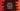# Python dictionary fromkeys method## Python dictionary fromkeys method:

fromkeys is an inbuilt method of Python dictionary and this method is used to create a new dictionary from a given sequence of elements.

In this post, we will learn how to use the fromkeys method with examples.

### Syntax of fromkeys method:

The fromkeys method is defined as like below:

``dict.fromkeys(s[, v])``

Where,

• s is the sequence of elements to use as keys in the dictionary.
• v is an optional parameter. This is the value to assign for each element of the dictionary. If we don’t provide this parameter, it assigns None.

#### Return value of fromkeys:

The fromkeys method creates a new dictionary and returns that dictionary. It uses the elements of the sequence passed as the first parameter as the keys of the dictionary.

If the value of v is given, it assigns this as the value of each element in the dictionary.

Let’s try fromKeys method with different examples:

### Example 1: Create a dictionary from a sequence:

Let’s use fromkeys with a sequence to create a dictionary:

``````s = {'one', 'two', 'three', 'four'}

d = dict.fromkeys(s)

print(d)``````

In this example, it will create a dictionary by using the elements of s as the keys. If you run this program, it will print the below output:

``{'three': None, 'four': None, 'one': None, 'two': None}``

As you can see, the keys of the dictionary are picked from the sequence s and the values are assigned as None.

### Example 2: Create a dictionary from a sequence and value:

Let’s try to create a dictionary by using a sequence as the keys and a value for each. We will modify the above example and provide a value to fromkeys:

``````s = {'one', 'two', 'three', 'four'}

d = dict.fromkeys(s, 0)

print(d)``````

It will print:

``{'two': 0, 'four': 0, 'one': 0, 'three': 0}``

As you can see, the value of each of the items of the dictionary are assigned as 0.

### Things to consider while using it with mutable values:

Note that fromkeys method assigns a reference of the value to each key in the dictionary. So, if the value is mutable and if we make any change to the value, the change reflects to all values in that dictionary.

For example,

``````s = {'one', 'two', 'three', 'four'}
v = 

d = dict.fromkeys(s, v)

print(d)

v.append(2)

print(d)``````

In this example, s is used as the keys of the dictionary and v is a list and it is used as the values of the dictionary keys.

It is printing the dictionary and appends 2 to the list v. It prints the dictionary again, but it will modify the values of each key:

``````{'two': , 'one': , 'four': , 'three': }
{'two': [0, 2], 'one': [0, 2], 'four': [0, 2], 'three': [0, 2]}``````

As you can see, the values are changed once we changed the list used as value. You can use fromkeys to create a dictionary with mutable values only if you are sure that the value won’t be changed. Or, you can use list comprehension to create the dictionary:

``````s = {'one', 'two', 'three', 'four'}
v = 

d = {k: list(v) for k in s}

print(d)

v.append(2)

print(d)``````

It will not change the values, even though we are making change to v. It will print:

``````{'four': , 'two': , 'one': , 'three': }
{'four': , 'two': , 'one': , 'three': }``````